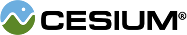#BlendEquation

#### BlendEquation() Scene/BlendEquation.js 15

Determines how two pixels' values are combined.

### Members

#### staticconstantCesium.BlendEquation.ADD : Number Scene/BlendEquation.js 22

Pixel values are added componentwise. This is used in additive blending for translucency.

#### staticconstantCesium.BlendEquation.REVERSE_SUBTRACT : Number Scene/BlendEquation.js 38

Pixel values are subtracted componentwise (destination - source).

#### staticconstantCesium.BlendEquation.SUBTRACT : Number Scene/BlendEquation.js 30

Pixel values are subtracted componentwise (source - destination). This is used in alpha blending for translucency.